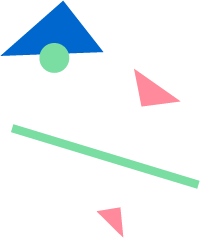# Between

Returns whether a value lies in the range between two other values.

## Usage

`Between(value, lower bound, upper bound)`

value (required)  The input value to check.
lower bound (required) The lower bound of the range to check.
upper bound (required) The upper bound of the range to check.

## Example

`Between([Invoice Date], MakeDate(2015, 1, 1), MakeDate(2020, 1, 1))`
• Returns TRUE if the date in the column name [Date] is between January 1, 2015 and January 1, 2020.
`Between([Sale Date], DateAdd("day", -7, Now()), Now())`
• Returns TRUE if a sale happened in the last 7 days